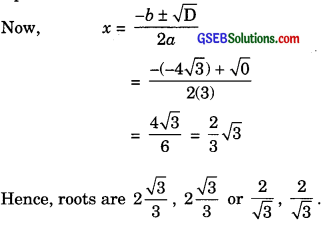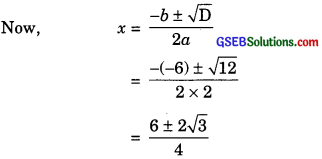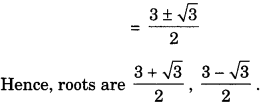# GSEB Solutions Class 10 Maths Chapter 4 Quadratic Equations Ex 4.4

Gujarat Board GSEB Solutions Class 10 Maths Chapter 4 Quadratic Equations Ex 4.4 Textbook Questions and Answers.

## Gujarat Board Textbook Solutions Class 10 Maths Chapter 4 Quadratic Equations Ex 4.4

Question 1.
Find the discriminant ofthe following quadratic equations. If the real roots exist, find them:
(i) 2x2 – 3x + 5 = 0
(ii) 3x2 – 4$$\sqrt { 3 }$$x + 4 = 0
(iii) 2x2 – 6x + 3 = 0
Solution:
(i) We have
2x2 – 3x + 5 = 0
Here, a = 2, b = – 3, c = 5
Now, D = b2 – 4ac
( – 3)2 – 4(2)(5)
= 9 – 40 = – 31
Since, D < 0
Therefore, the given quadratic equation has no real roots.

(ii) We have,
3x2 – 4$$\sqrt { 3 }$$x + 4 = 0
Here, a = 3, b = -4$$\sqrt { 3 }$$, c = 4
Now, D = b2 – 4ac
= ( – 4$$\sqrt { 3 }$$)2 – 4.3. 4
= 48 – 48 = 0
Since, D = 0
Therefore, the given quadratic equation has equal roots.2x2 – 6x + 3 = O
Here, a = 2, b = – 6, c = 3
Now, D = b2 – 4ac
= (- 6)2 – 4(2)(3)
= 36 – 24 = 12
Since, D > 0
Therefore, the given quadratic equation has real and district roots.Question 2.
Find the value of k for each of the following quadratic equations so that they have equal roots.
(i) 2x2 + kx + 3 = 0
(ii) kx (x – 2) + 6 = 0
Solution:
(i) We have,
2x2 + kx + 3 = 0
Here, a = 2, b = k,c = 3
Now, D = b2 – 4ac
(k)2 – 4(2)(3)
= k2 – 24
For equal roots,
D = 0
k2 – 24 = 0
k2 = 24
k = ± $$\sqrt { 2 }$$
k = ±$$\sqrt { 6×4 }$$
k = ±$$2\sqrt { 6 }$$

(ii) We have,
kx (x – 2) + 6 = 0
Here, a = k, b = – 2k, c = 6
Now, D = b2 – 4ac
D = ( – 2k)2 – 4(k)(6)
D = 4k2 – 24k
For equal roots,
D = 0
4k2 – 24k = 0
4k(k – 6) = 0
4k = 0 or k – 6 = 0
k = 0 or k = 6Question 3.
Is it possible to design a rectangular mango grove whose length is twice its breadth and the area is 800 m2? If so, find its length and breadth.
Solution:
Let the breadth of the rectangular mango grove be x m
So, length = 2x m
According to the question,
Area = 800 m2
2x (x) = 800
2x2 = 800
x2 = 400
x2 – 400 = 0
Here, a = 1, b = 0, c = – 400
so, D = b2 – 4ac
= – 4 x 1 x (- 400)
= 1600 > 0
Thus, designing the rectangular mango grove is possible.
x2 = 400
x =± $$\sqrt { 400 }$$ = ± 20
x = + 20 and – 20
x = – 20 is not possible.
So, x = 20
Hence, breadth of the rectangular mango grove is 20 m and length is 40 m.Question 4.
Is the following situation possible? If so, determine their present ages. The sum of the ages of two friends is 20 years. Four years ago, the product of their ages in years was 48.
Solution:
Let the present age of first friend be x years.
So, present age of other be (20 – x),
4 years ago,
Age of first friend = (x – 4) years
and age of second friend = (20 – x – 4) years
= (16 – x) years
According to question,
(x – 4) (16 – x) = 48
= 16x – x2 – 64 + 4x = 48
– x2 + 20x – 112 = 0
x2 – 20x + 112 = 0
Here, a = 1, b = – 20, c = 112
D = b2 – 4ac
= ( – 20)2 – 4(1)(112)
=400 – 448 = – 48
Since, D < 0
So, the given quadratic equation has no real roots and hence the given situation is not possible.